Скачать презентацию CHAPTER 2 COST CONCEPTS AND THE ECONOMIC ENVIRONMENT

6e44b352c380c5fd0571d213b1076a5a.ppt

• Количество слайдов: 45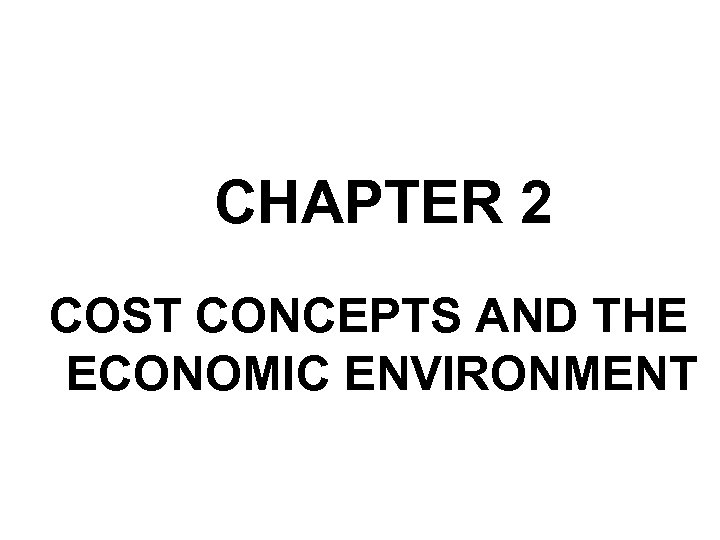CHAPTER 2 COST CONCEPTS AND THE ECONOMIC ENVIRONMENT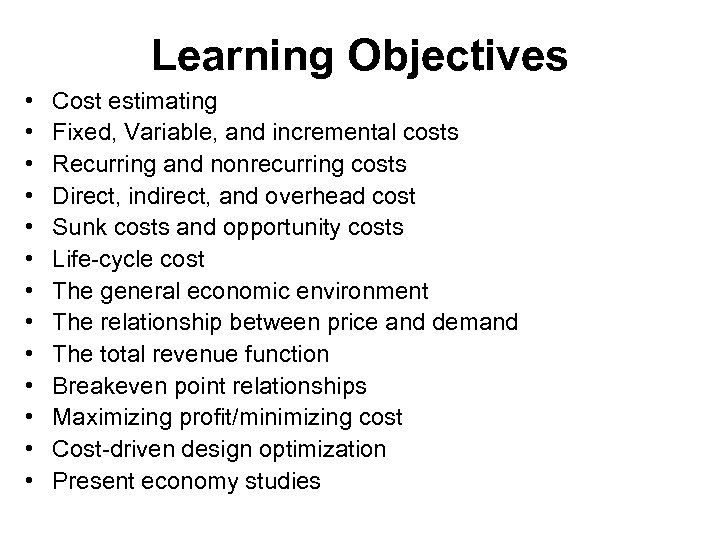Learning Objectives • • • • Cost estimating Fixed, Variable, and incremental costs Recurring and nonrecurring costs Direct, indirect, and overhead cost Sunk costs and opportunity costs Life-cycle cost The general economic environment The relationship between price and demand The total revenue function Breakeven point relationships Maximizing profit/minimizing cost Cost-driven design optimization Present economy studies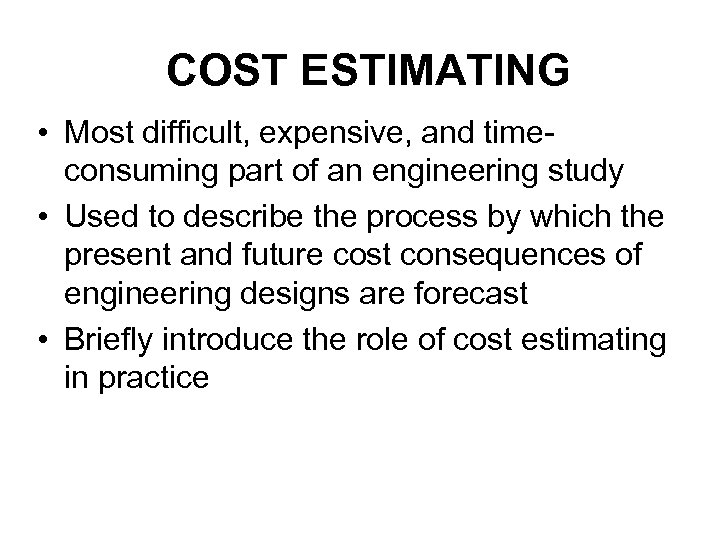COST ESTIMATING • Most difficult, expensive, and timeconsuming part of an engineering study • Used to describe the process by which the present and future cost consequences of engineering designs are forecast • Briefly introduce the role of cost estimating in practice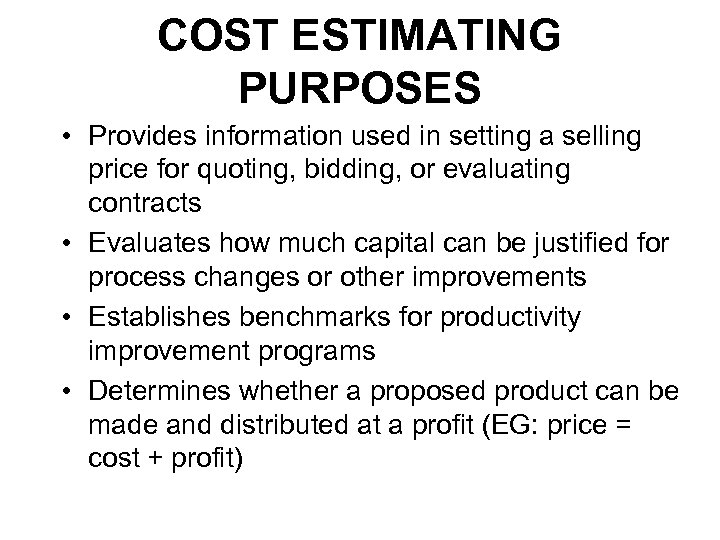COST ESTIMATING PURPOSES • Provides information used in setting a selling price for quoting, bidding, or evaluating contracts • Evaluates how much capital can be justified for process changes or other improvements • Establishes benchmarks for productivity improvement programs • Determines whether a proposed product can be made and distributed at a profit (EG: price = cost + profit)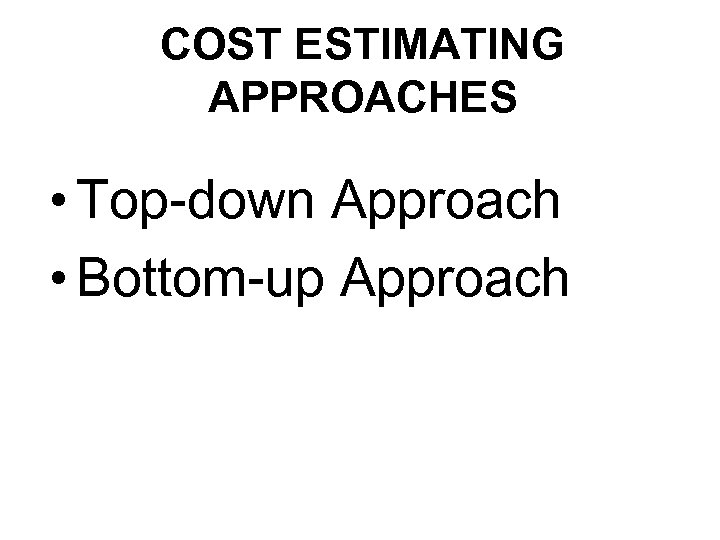COST ESTIMATING APPROACHES • Top-down Approach • Bottom-up Approach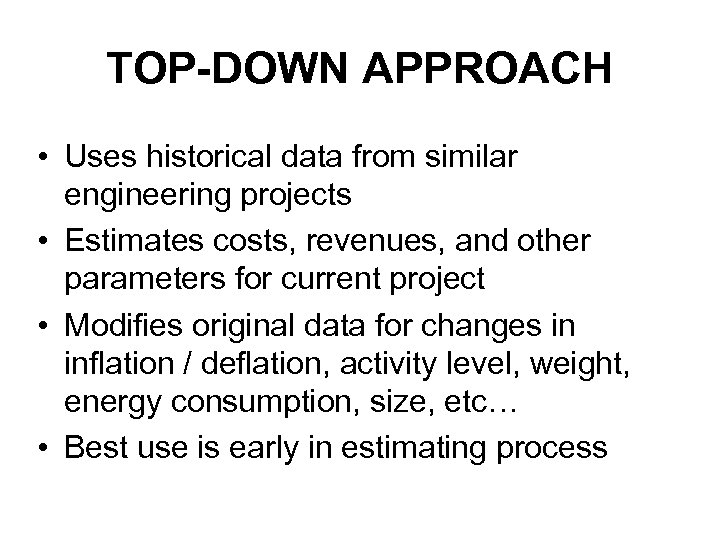TOP-DOWN APPROACH • Uses historical data from similar engineering projects • Estimates costs, revenues, and other parameters for current project • Modifies original data for changes in inflation / deflation, activity level, weight, energy consumption, size, etc… • Best use is early in estimating process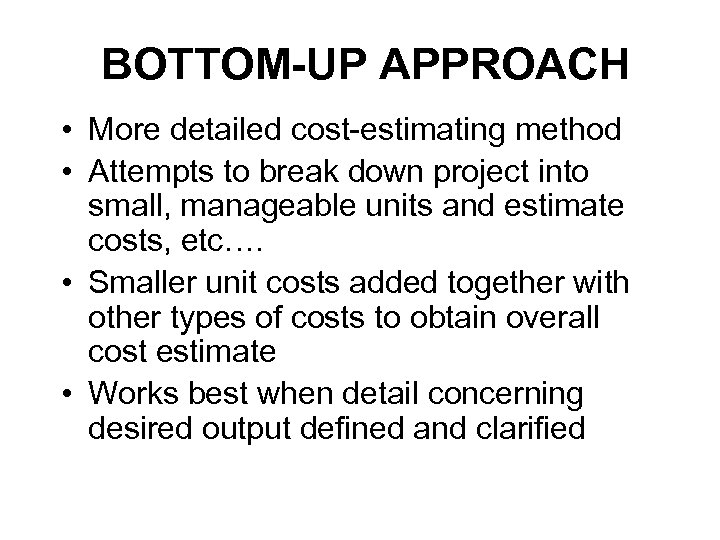BOTTOM-UP APPROACH • More detailed cost-estimating method • Attempts to break down project into small, manageable units and estimate costs, etc…. • Smaller unit costs added together with other types of costs to obtain overall cost estimate • Works best when detail concerning desired output defined and clarified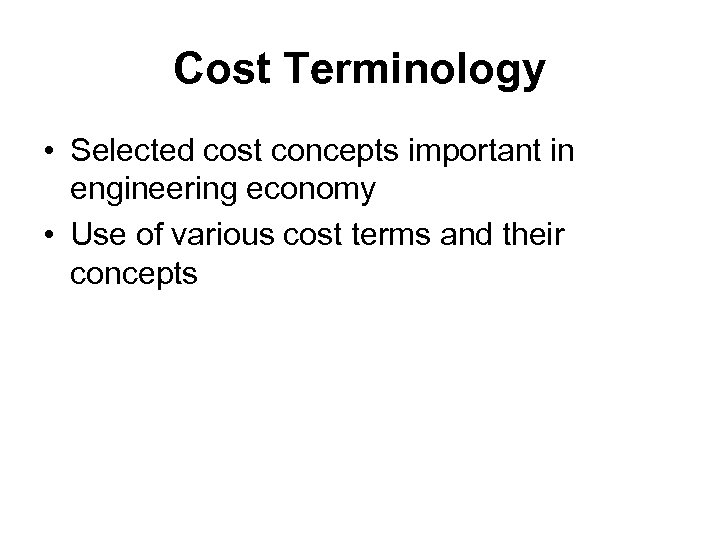Cost Terminology • Selected cost concepts important in engineering economy • Use of various cost terms and their concepts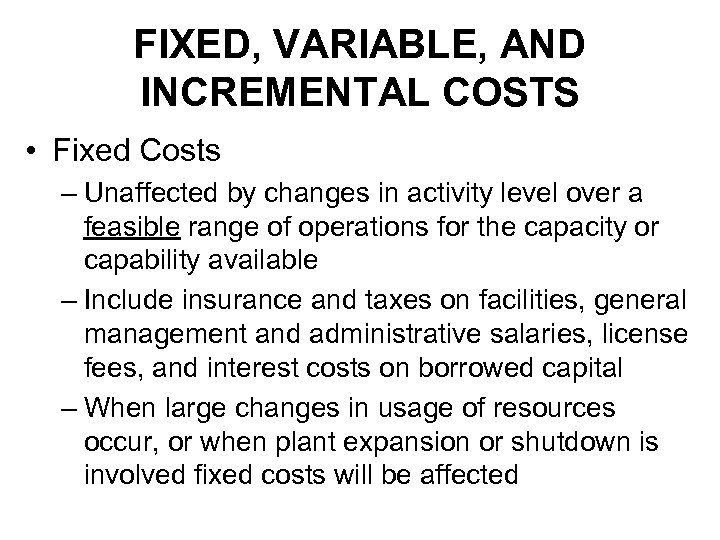FIXED, VARIABLE, AND INCREMENTAL COSTS • Fixed Costs – Unaffected by changes in activity level over a feasible range of operations for the capacity or capability available – Include insurance and taxes on facilities, general management and administrative salaries, license fees, and interest costs on borrowed capital – When large changes in usage of resources occur, or when plant expansion or shutdown is involved fixed costs will be affected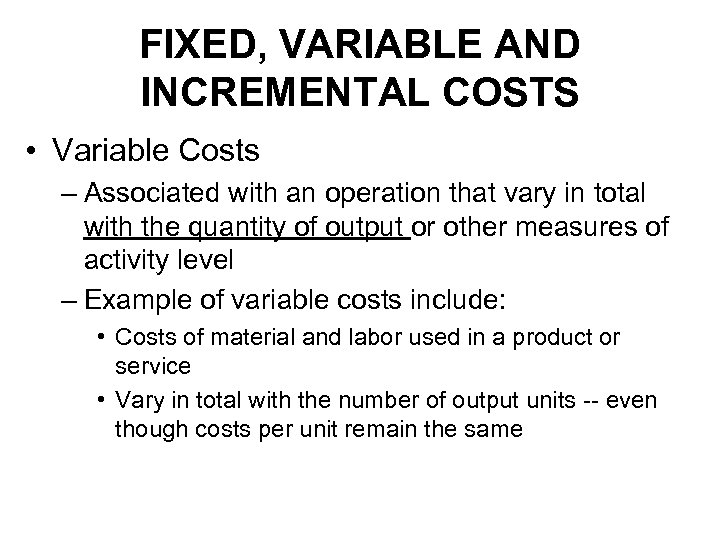FIXED, VARIABLE AND INCREMENTAL COSTS • Variable Costs – Associated with an operation that vary in total with the quantity of output or other measures of activity level – Example of variable costs include: • Costs of material and labor used in a product or service • Vary in total with the number of output units -- even though costs per unit remain the same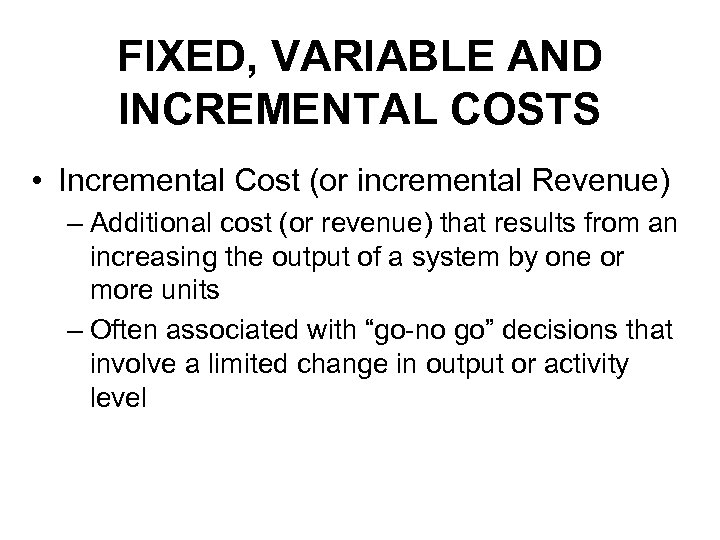FIXED, VARIABLE AND INCREMENTAL COSTS • Incremental Cost (or incremental Revenue) – Additional cost (or revenue) that results from an increasing the output of a system by one or more units – Often associated with “go-no go” decisions that involve a limited change in output or activity level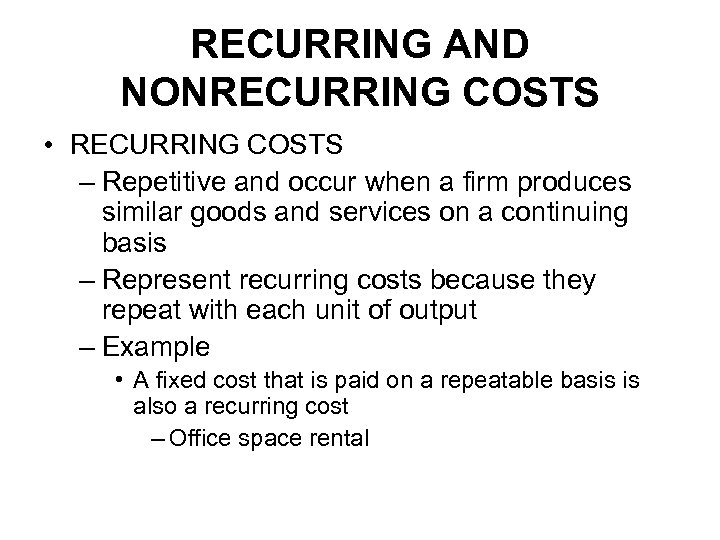RECURRING AND NONRECURRING COSTS • RECURRING COSTS – Repetitive and occur when a firm produces similar goods and services on a continuing basis – Represent recurring costs because they repeat with each unit of output – Example • A fixed cost that is paid on a repeatable basis is also a recurring cost – Office space rental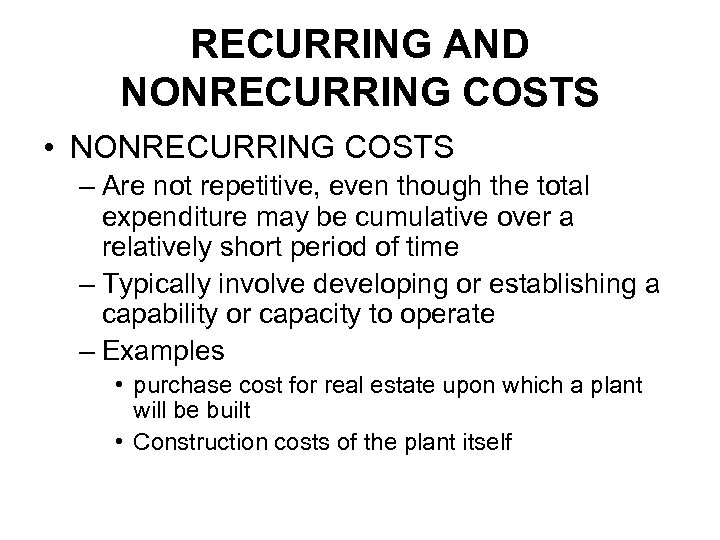RECURRING AND NONRECURRING COSTS • NONRECURRING COSTS – Are not repetitive, even though the total expenditure may be cumulative over a relatively short period of time – Typically involve developing or establishing a capability or capacity to operate – Examples • purchase cost for real estate upon which a plant will be built • Construction costs of the plant itself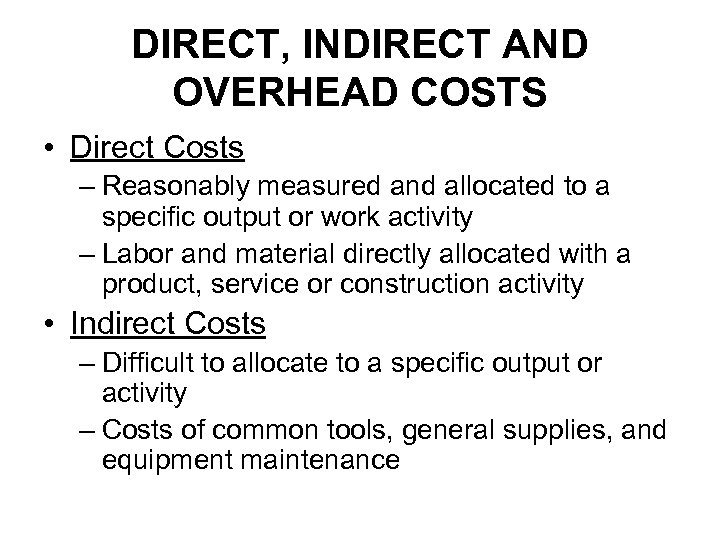DIRECT, INDIRECT AND OVERHEAD COSTS • Direct Costs – Reasonably measured and allocated to a specific output or work activity – Labor and material directly allocated with a product, service or construction activity • Indirect Costs – Difficult to allocate to a specific output or activity – Costs of common tools, general supplies, and equipment maintenance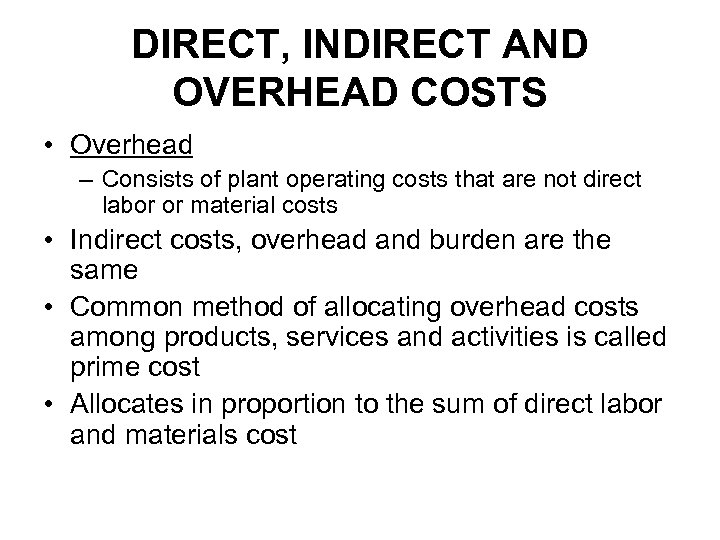DIRECT, INDIRECT AND OVERHEAD COSTS • Overhead – Consists of plant operating costs that are not direct labor or material costs • Indirect costs, overhead and burden are the same • Common method of allocating overhead costs among products, services and activities is called prime cost • Allocates in proportion to the sum of direct labor and materials cost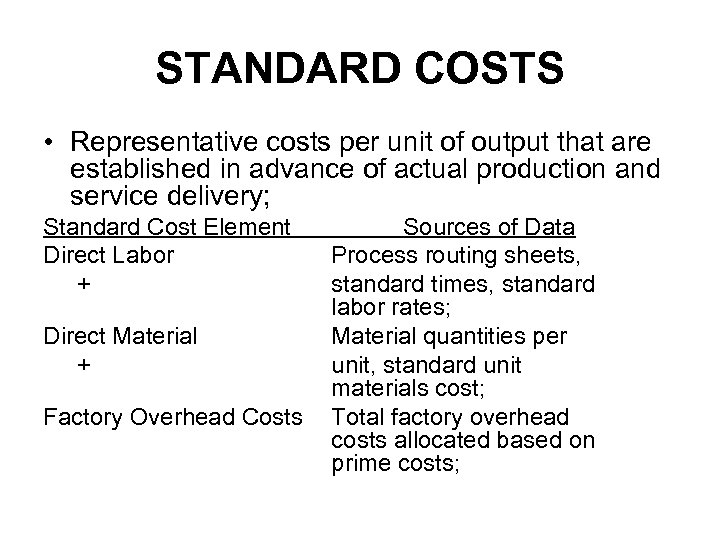STANDARD COSTS • Representative costs per unit of output that are established in advance of actual production and service delivery; Standard Cost Element Direct Labor + Direct Material + Factory Overhead Costs Sources of Data Process routing sheets, standard times, standard labor rates; Material quantities per unit, standard unit materials cost; Total factory overhead costs allocated based on prime costs;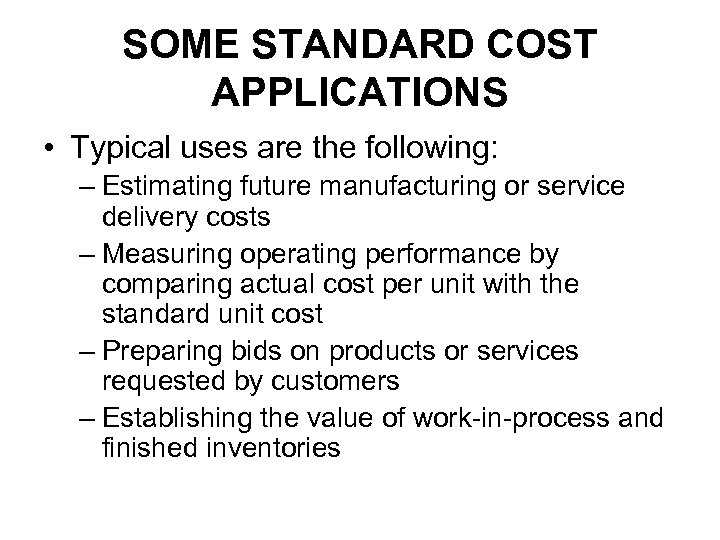SOME STANDARD COST APPLICATIONS • Typical uses are the following: – Estimating future manufacturing or service delivery costs – Measuring operating performance by comparing actual cost per unit with the standard unit cost – Preparing bids on products or services requested by customers – Establishing the value of work-in-process and finished inventories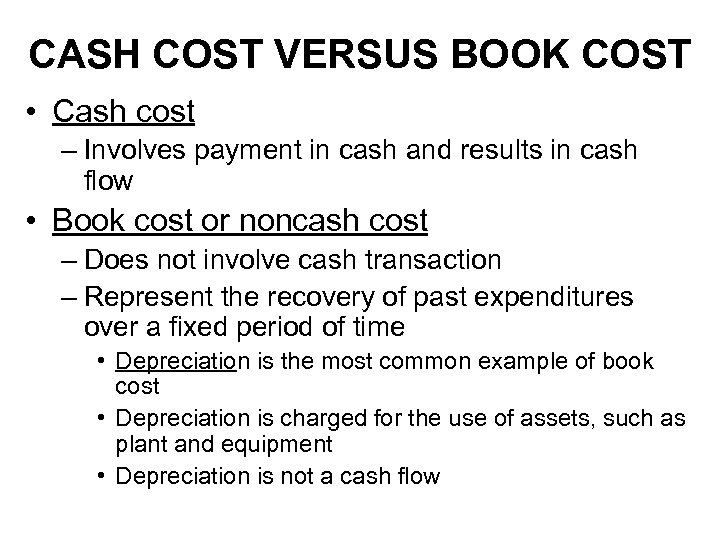CASH COST VERSUS BOOK COST • Cash cost – Involves payment in cash and results in cash flow • Book cost or noncash cost – Does not involve cash transaction – Represent the recovery of past expenditures over a fixed period of time • Depreciation is the most common example of book cost • Depreciation is charged for the use of assets, such as plant and equipment • Depreciation is not a cash flow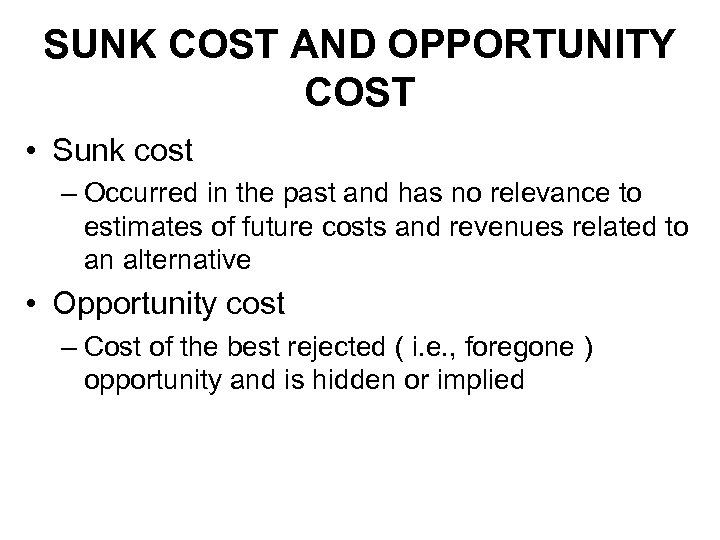SUNK COST AND OPPORTUNITY COST • Sunk cost – Occurred in the past and has no relevance to estimates of future costs and revenues related to an alternative • Opportunity cost – Cost of the best rejected ( i. e. , foregone ) opportunity and is hidden or implied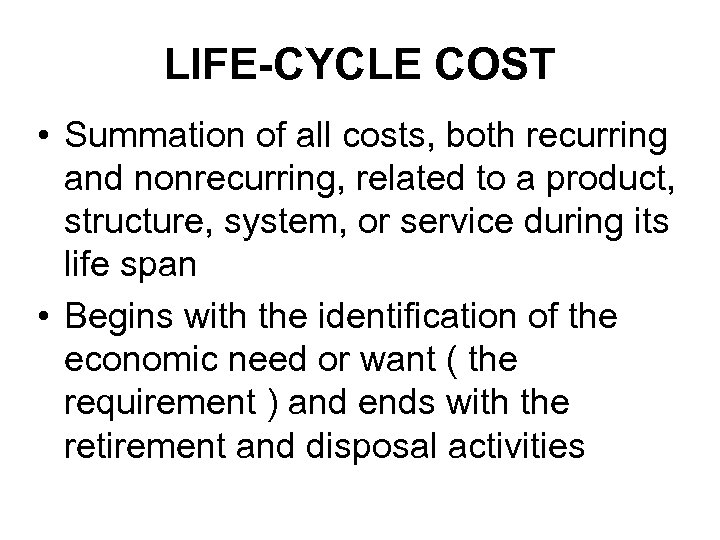LIFE-CYCLE COST • Summation of all costs, both recurring and nonrecurring, related to a product, structure, system, or service during its life span • Begins with the identification of the economic need or want ( the requirement ) and ends with the retirement and disposal activities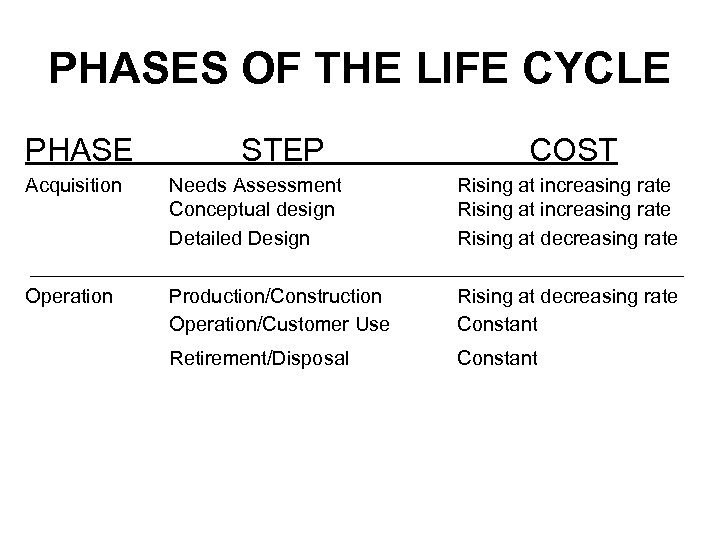PHASES OF THE LIFE CYCLE PHASE STEP COST Acquisition Needs Assessment Conceptual design Detailed Design Rising at increasing rate Rising at decreasing rate Operation Production/Construction Operation/Customer Use Rising at decreasing rate Constant Retirement/Disposal Constant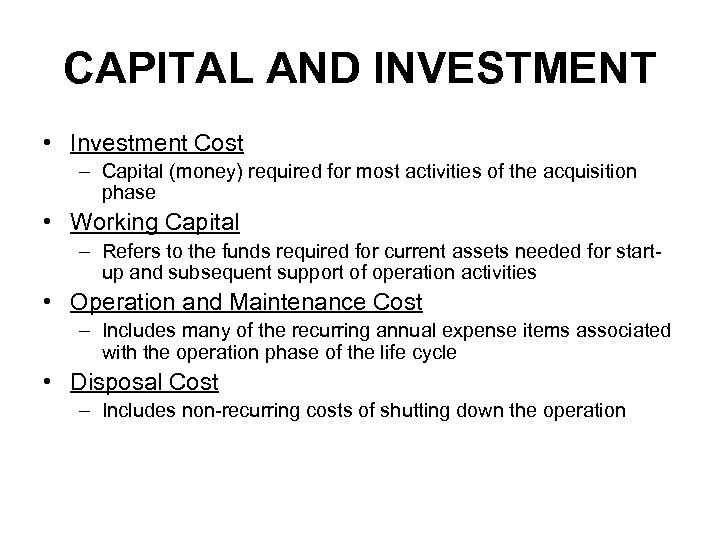CAPITAL AND INVESTMENT • Investment Cost – Capital (money) required for most activities of the acquisition phase • Working Capital – Refers to the funds required for current assets needed for startup and subsequent support of operation activities • Operation and Maintenance Cost – Includes many of the recurring annual expense items associated with the operation phase of the life cycle • Disposal Cost – Includes non-recurring costs of shutting down the operationGENERAL FORMULA • Life Cycle Cost = Investment Costs + Working Capital + O&M Costs+ Disposal Costs or Salvage Value (if any)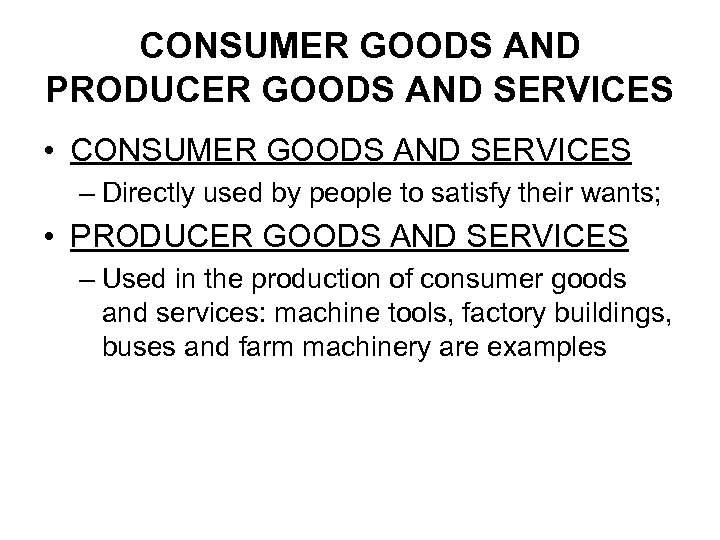CONSUMER GOODS AND PRODUCER GOODS AND SERVICES • CONSUMER GOODS AND SERVICES – Directly used by people to satisfy their wants; • PRODUCER GOODS AND SERVICES – Used in the production of consumer goods and services: machine tools, factory buildings, buses and farm machinery are examples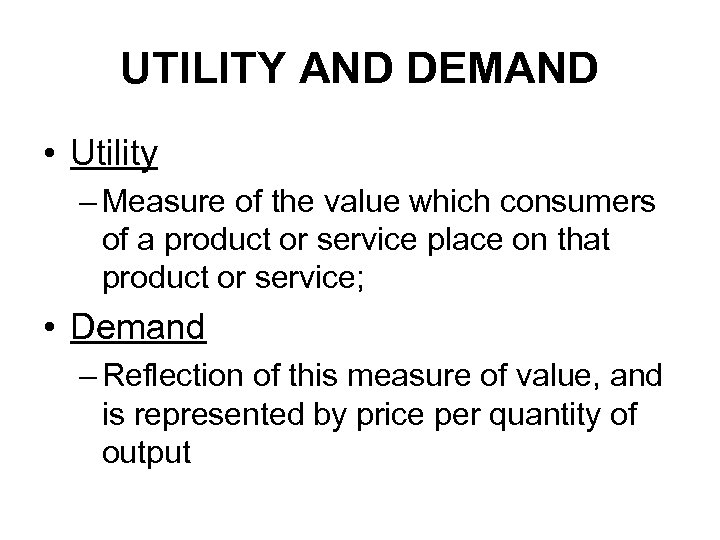UTILITY AND DEMAND • Utility – Measure of the value which consumers of a product or service place on that product or service; • Demand – Reflection of this measure of value, and is represented by price per quantity of output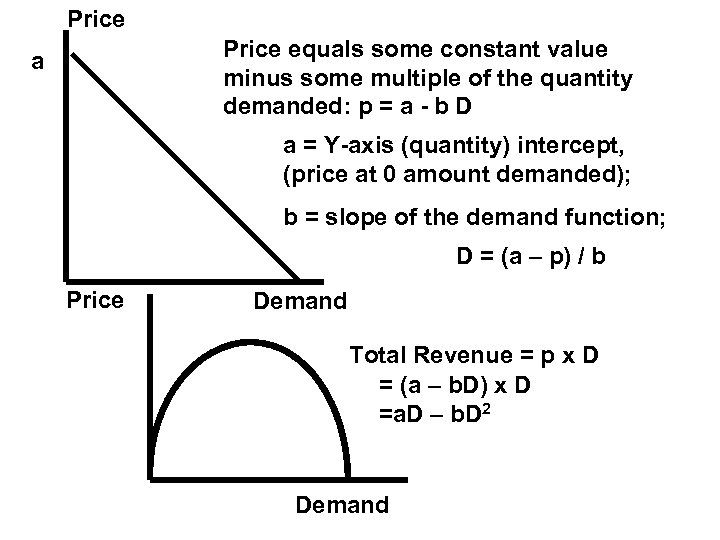Price equals some constant value minus some multiple of the quantity demanded: p = a - b D a a = Y-axis (quantity) intercept, (price at 0 amount demanded); b = slope of the demand function; D = (a – p) / b Price Demand Total Revenue = p x D = (a – b. D) x D =a. D – b. D 2 Demand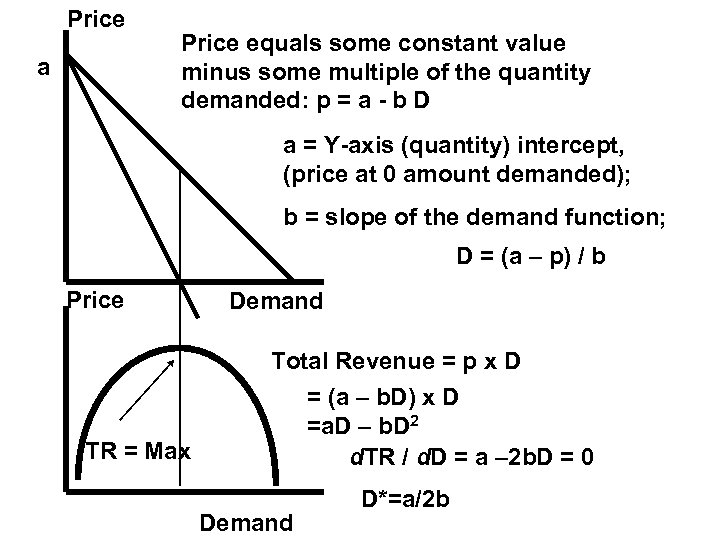Price a Price equals some constant value minus some multiple of the quantity demanded: p = a - b D a = Y-axis (quantity) intercept, (price at 0 amount demanded); b = slope of the demand function; D = (a – p) / b Price TR = Max Demand Total Revenue = p x D = (a – b. D) x D =a. D – b. D 2 d. TR / d. D = a – 2 b. D = 0 Demand D*=a/2 b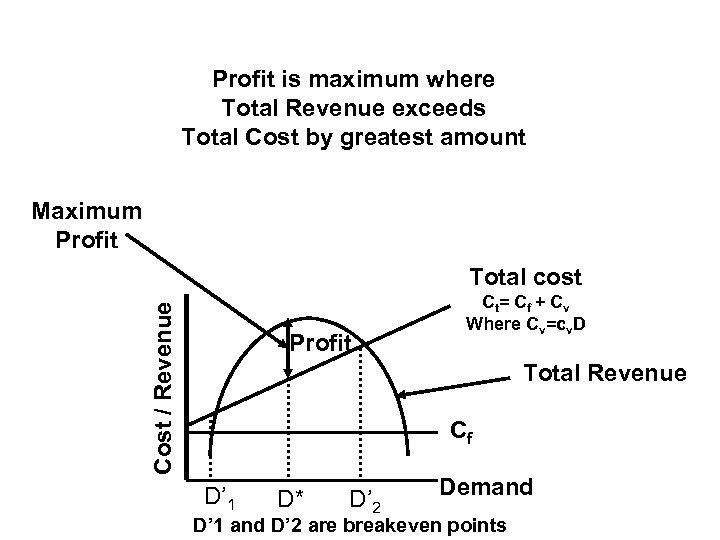Profit is maximum where Total Revenue exceeds Total Cost by greatest amount Maximum Profit Cost / Revenue Total cost Profit Ct = Cf + Cv Where Cv=cv. D Total Revenue Cf D’ 1 D* D’ 2 Demand D’ 1 and D’ 2 are breakeven points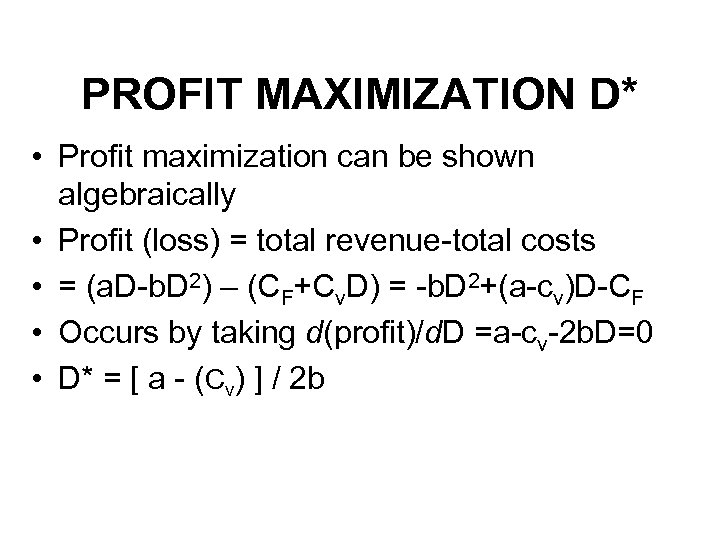PROFIT MAXIMIZATION D* • Profit maximization can be shown algebraically • Profit (loss) = total revenue-total costs • = (a. D-b. D 2) – (CF+Cv. D) = -b. D 2+(a-cv)D-CF • Occurs by taking d(profit)/d. D =a-cv-2 b. D=0 • D* = [ a - (Cv) ] / 2 b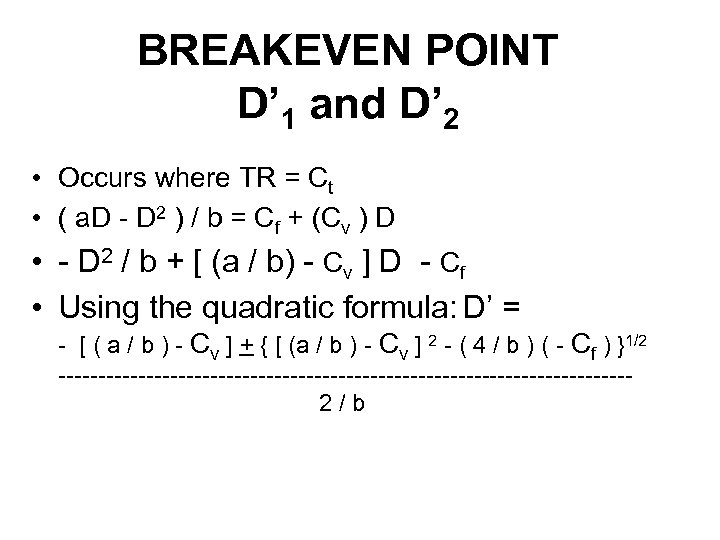BREAKEVEN POINT D’ 1 and D’ 2 • Occurs where TR = Ct • ( a. D - D 2 ) / b = Cf + (Cv ) D • - D 2 / b + [ (a / b) - Cv ] D - Cf • Using the quadratic formula: D’ = - [ ( a / b ) - Cv ] + { [ (a / b ) - Cv ] 2 - ( 4 / b ) ( - Cf ) }1/2 ------------------------------------2/b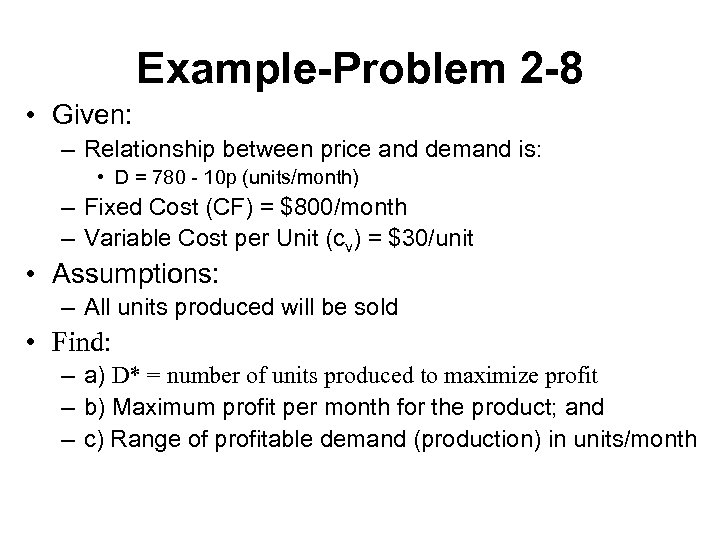Example-Problem 2 -8 • Given: – Relationship between price and demand is: • D = 780 - 10 p (units/month) – Fixed Cost (CF) = \$800/month – Variable Cost per Unit (cv) = \$30/unit • Assumptions: – All units produced will be sold • Find: – a) D* = number of units produced to maximize profit – b) Maximum profit per month for the product; and – c) Range of profitable demand (production) in units/month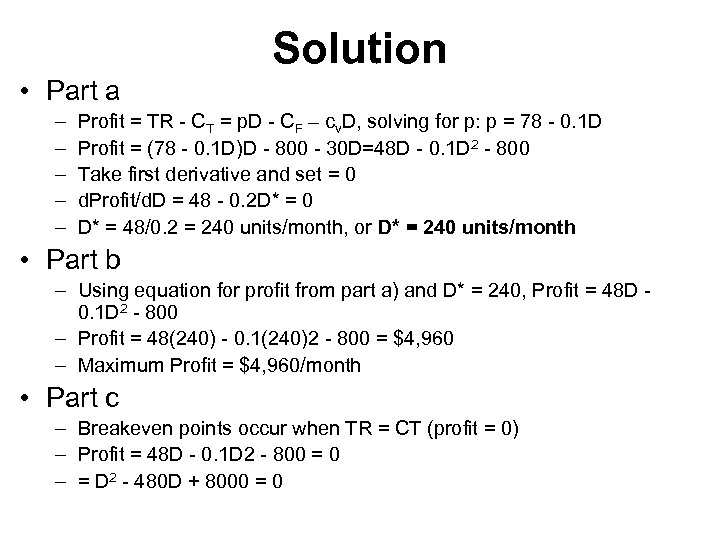Solution • Part a – – – Profit = TR - CT = p. D - CF – cv. D, solving for p: p = 78 - 0. 1 D Profit = (78 - 0. 1 D)D - 800 - 30 D=48 D - 0. 1 D 2 - 800 Take first derivative and set = 0 d. Profit/d. D = 48 - 0. 2 D* = 0 D* = 48/0. 2 = 240 units/month, or D* = 240 units/month • Part b – Using equation for profit from part a) and D* = 240, Profit = 48 D 0. 1 D 2 - 800 – Profit = 48(240) - 0. 1(240)2 - 800 = \$4, 960 – Maximum Profit = \$4, 960/month • Part c – Breakeven points occur when TR = CT (profit = 0) – Profit = 48 D - 0. 1 D 2 - 800 = 0 – = D 2 - 480 D + 8000 = 0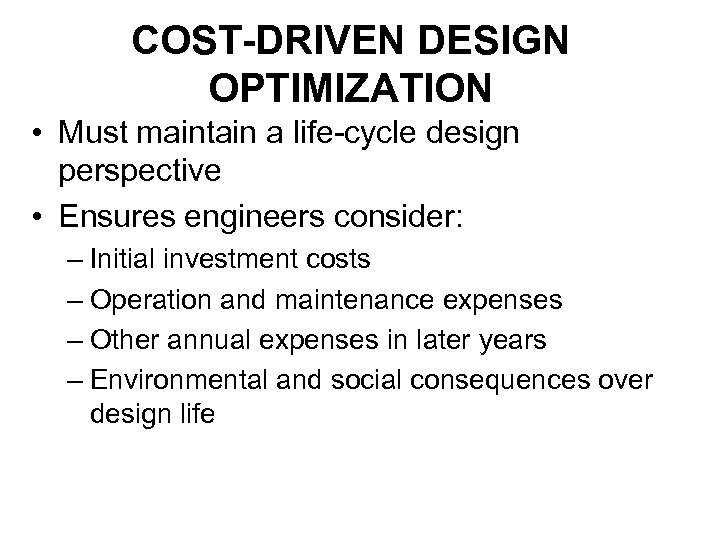COST-DRIVEN DESIGN OPTIMIZATION • Must maintain a life-cycle design perspective • Ensures engineers consider: – Initial investment costs – Operation and maintenance expenses – Other annual expenses in later years – Environmental and social consequences over design life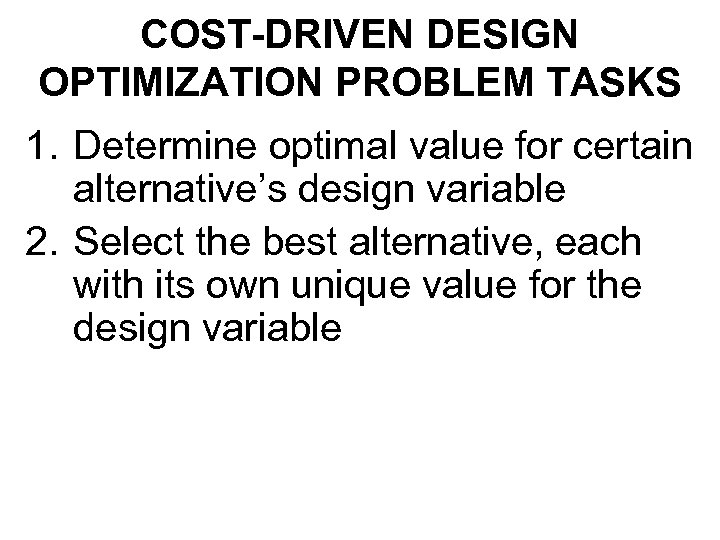COST-DRIVEN DESIGN OPTIMIZATION PROBLEM TASKS 1. Determine optimal value for certain alternative’s design variable 2. Select the best alternative, each with its own unique value for the design variable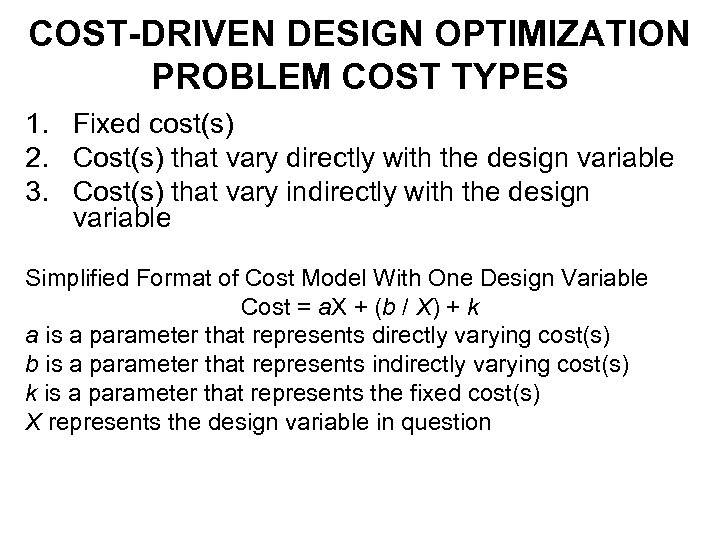COST-DRIVEN DESIGN OPTIMIZATION PROBLEM COST TYPES 1. Fixed cost(s) 2. Cost(s) that vary directly with the design variable 3. Cost(s) that vary indirectly with the design variable Simplified Format of Cost Model With One Design Variable Cost = a. X + (b / X) + k a is a parameter that represents directly varying cost(s) b is a parameter that represents indirectly varying cost(s) k is a parameter that represents the fixed cost(s) X represents the design variable in question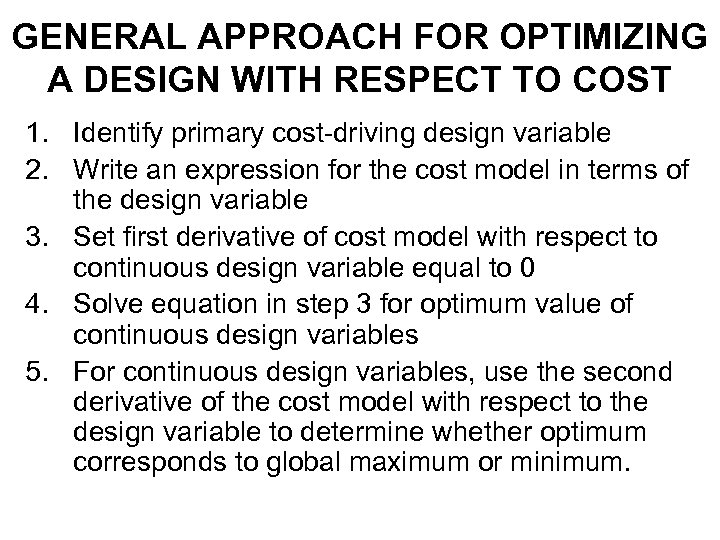GENERAL APPROACH FOR OPTIMIZING A DESIGN WITH RESPECT TO COST 1. Identify primary cost-driving design variable 2. Write an expression for the cost model in terms of the design variable 3. Set first derivative of cost model with respect to continuous design variable equal to 0 4. Solve equation in step 3 for optimum value of continuous design variables 5. For continuous design variables, use the second derivative of the cost model with respect to the design variable to determine whether optimum corresponds to global maximum or minimum.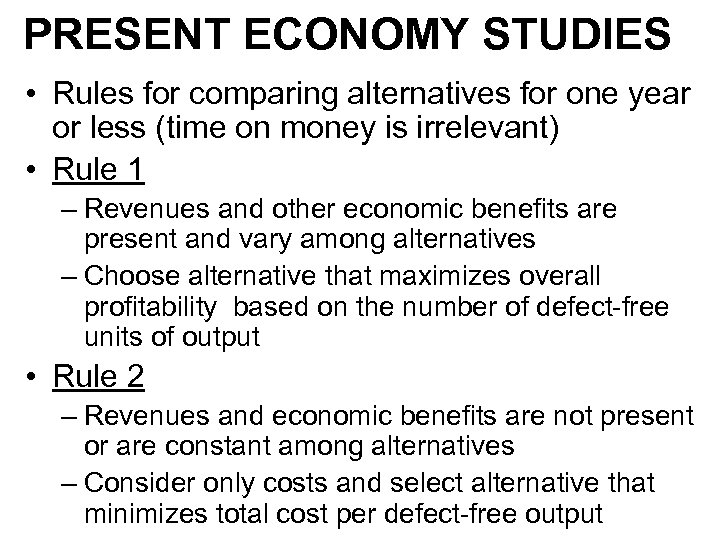PRESENT ECONOMY STUDIES • Rules for comparing alternatives for one year or less (time on money is irrelevant) • Rule 1 – Revenues and other economic benefits are present and vary among alternatives – Choose alternative that maximizes overall profitability based on the number of defect-free units of output • Rule 2 – Revenues and economic benefits are not present or are constant among alternatives – Consider only costs and select alternative that minimizes total cost per defect-free output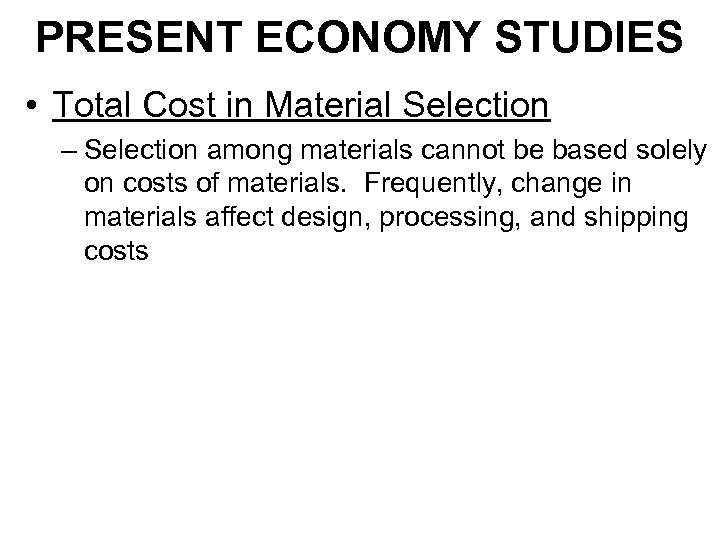PRESENT ECONOMY STUDIES • Total Cost in Material Selection – Selection among materials cannot be based solely on costs of materials. Frequently, change in materials affect design, processing, and shipping costs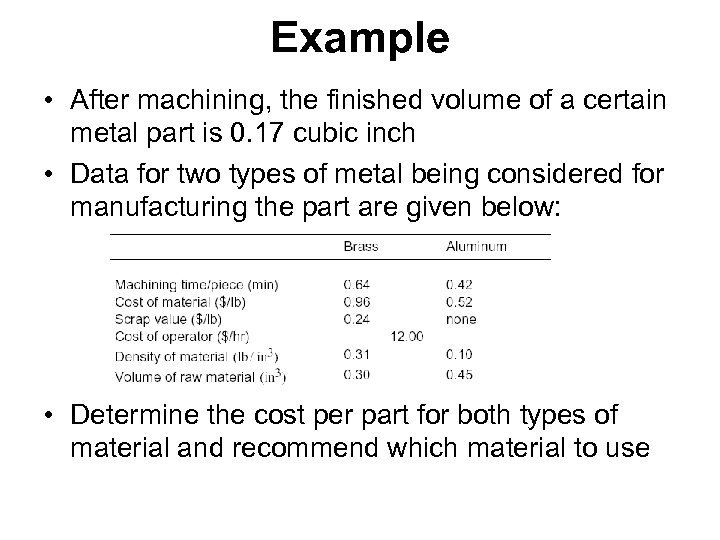Example • After machining, the finished volume of a certain metal part is 0. 17 cubic inch • Data for two types of metal being considered for manufacturing the part are given below: • Determine the cost per part for both types of material and recommend which material to use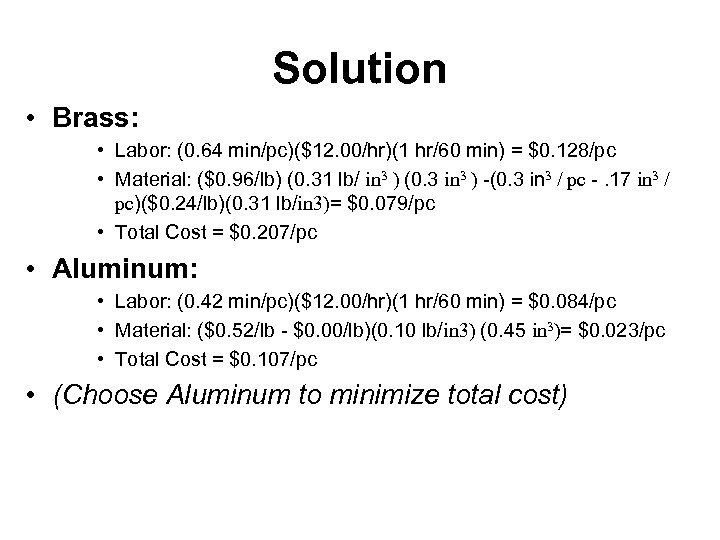Solution • Brass: • Labor: (0. 64 min/pc)(\$12. 00/hr)(1 hr/60 min) = \$0. 128/pc • Material: (\$0. 96/lb) (0. 31 lb/ in 3 ) (0. 3 in 3 ) -(0. 3 in 3 / pc -. 17 in 3 / pc)(\$0. 24/lb)(0. 31 lb/in 3)= \$0. 079/pc • Total Cost = \$0. 207/pc • Aluminum: • Labor: (0. 42 min/pc)(\$12. 00/hr)(1 hr/60 min) = \$0. 084/pc • Material: (\$0. 52/lb - \$0. 00/lb)(0. 10 lb/in 3) (0. 45 in 3)= \$0. 023/pc • Total Cost = \$0. 107/pc • (Choose Aluminum to minimize total cost)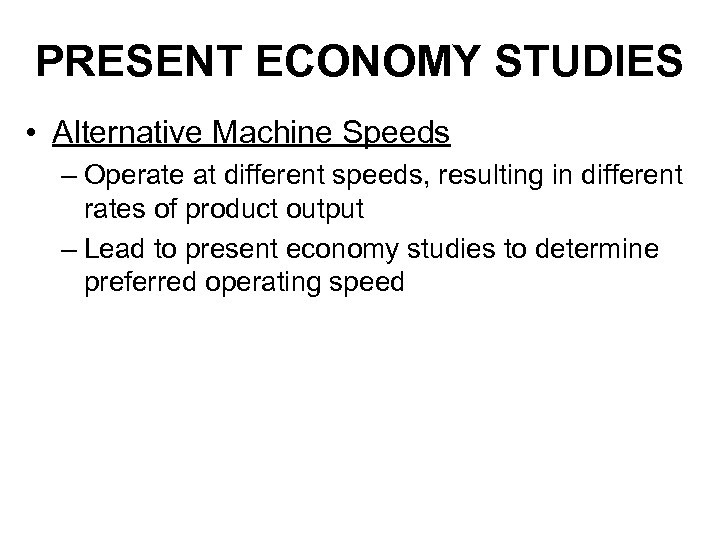PRESENT ECONOMY STUDIES • Alternative Machine Speeds – Operate at different speeds, resulting in different rates of product output – Lead to present economy studies to determine preferred operating speed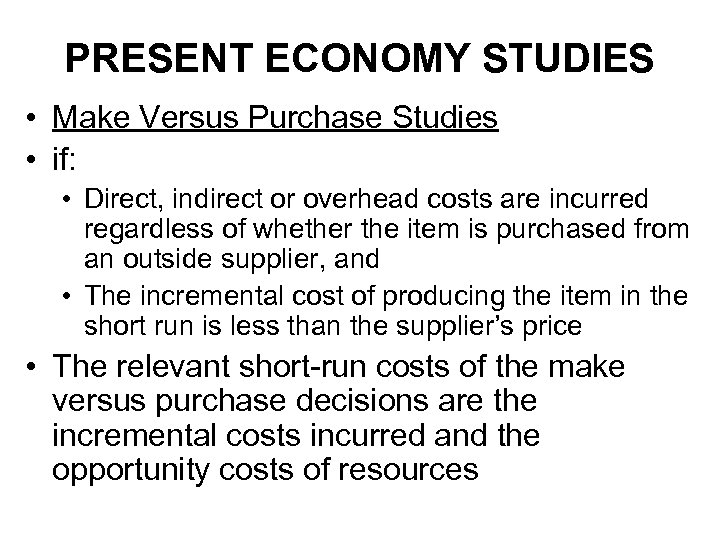PRESENT ECONOMY STUDIES • Make Versus Purchase Studies • if: • Direct, indirect or overhead costs are incurred regardless of whether the item is purchased from an outside supplier, and • The incremental cost of producing the item in the short run is less than the supplier’s price • The relevant short-run costs of the make versus purchase decisions are the incremental costs incurred and the opportunity costs of resources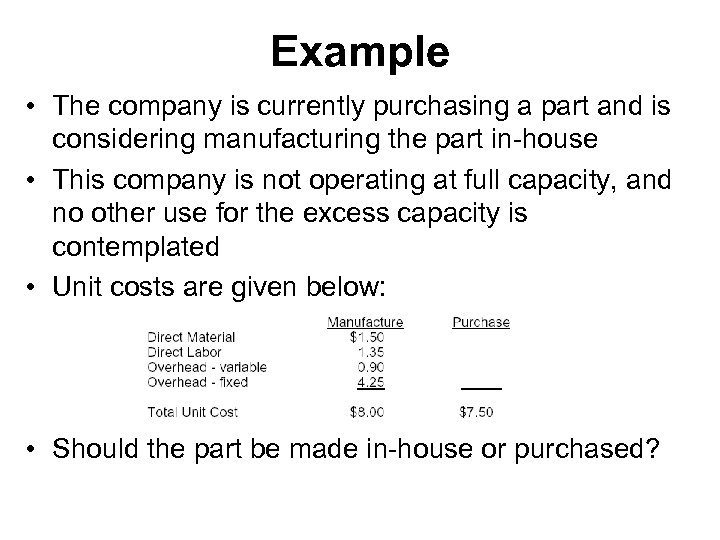Example • The company is currently purchasing a part and is considering manufacturing the part in-house • This company is not operating at full capacity, and no other use for the excess capacity is contemplated • Unit costs are given below: • Should the part be made in-house or purchased?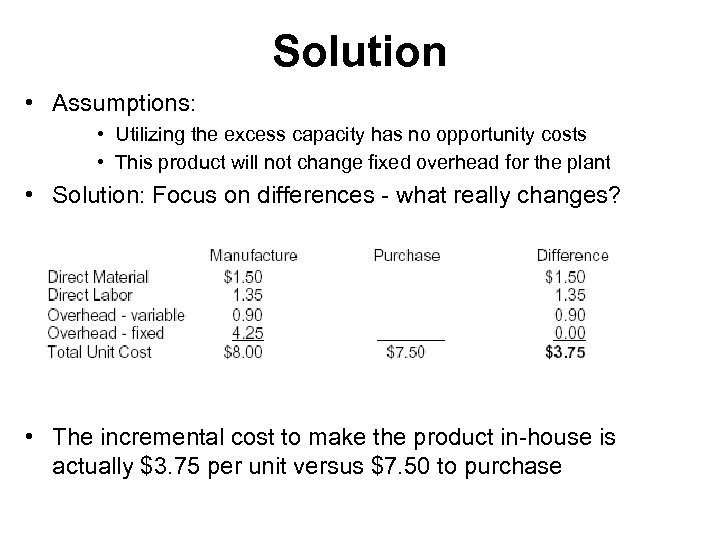Solution • Assumptions: • Utilizing the excess capacity has no opportunity costs • This product will not change fixed overhead for the plant • Solution: Focus on differences - what really changes? • The incremental cost to make the product in-house is actually \$3. 75 per unit versus \$7. 50 to purchase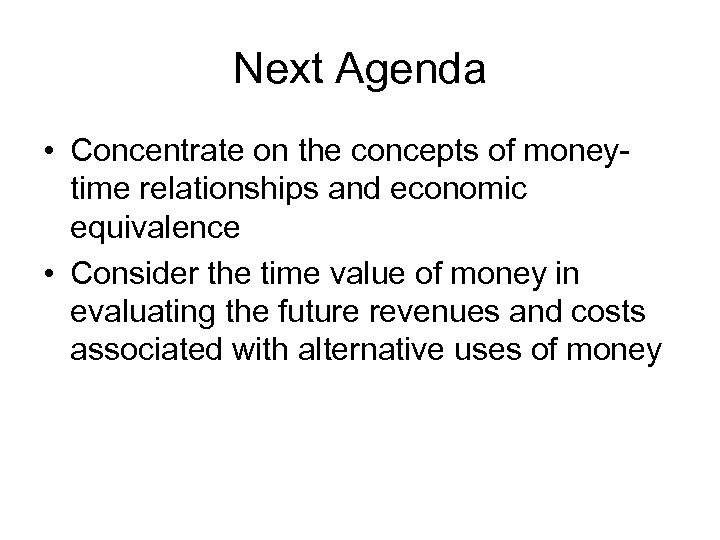Next Agenda • Concentrate on the concepts of moneytime relationships and economic equivalence • Consider the time value of money in evaluating the future revenues and costs associated with alternative uses of money